# A SIFT Description Approach for Non-Uniform Illumination and Other Invariants

A SIFT Description Approach for Non-Uniform Illumination and Other Invariants
Faculty of Engineering, Mahasarakham University, Mahasarakham 44150, Thailand

Corresponding Author Email:
wor.nui@gmail.com
Page:
533-539
|
DOI:
https://doi.org/10.18280/isi.260603
6 September 2021
|
Accepted:
19 November 2021
|
Published:
27 December 2021
| Citation

OPEN ACCESS

Abstract:

The new improvement keypoint description technique of image-based recognition for rotation, viewpoint and non-uniform illumination situations is presented. The technique is relatively simple based on two procedures, i.e., the keypoint detection and the keypoint description procedure. The keypoint detection procedure is based on the SIFT approach, Top-Hat filtering, morphological operations and average filtering approach. Where this keypoint detection procedure can segment the targets from uneven illumination particle images. While the keypoint description procedures are described and implemented using the Hu moment invariants. Where the central moments are being unchanged under image translations. The sensitivity, accuracy and precision rate of data sets were evaluated and compared. The data set are provided by color image database with variants uniform and non-uniform illumination, viewpoint and rotation changes. The evaluative results show that the approach is superior to the other SIFTs in terms of uniform illumination, non-uniform illumination and other situations. Additionally, the paper demonstrates the high sensitivity of 100%, high accuracy of 83.33% and high precision rate of 80.00%. Comparisons to other SIFT approaches are also included.

Keywords:

keypoint description, matching, image moment, SIFT, invariants

1. Introduction

Nowadays, computer vision has become one of the most cited topics and challenges in century . However, computer vision leads to many challenging problems in the undesirable situations and uncontrollable factors such as the light sources on partial surface, the different absorption and non-uniform light field illumination. Therefore, the several approaches have been presented to overcome these problems . For example, the elementary characteristics are hopefully invariant over the different locality, distinctive, quantity, invariant and illumination situations. But these feature descriptors robust lead to photometric changes [3-6]. Recently, the Scale Invariance Feature Transform (SIFT)  is proposed a local feature detection and description approach for two-dimensional image as the desirable situations and controllable factors.

The survey on local scale invariant features are concisely discussed. Affine-SIFT known as ASIFT , which follows affine transformation parameters to correct images and purposes to oppose intensive affine issues. Moreover, the SURF, CSIFT, PCA-SIFT, GSIFT and ASIFT are proposed . These approaches are the subclass of the SIFT’s family. The performance of the SIFT techniques do not perform well in case the rotating, scaling, affine, blur and illumination invariance. Therefore, the SIFT techniques are also necessary for precondition to untangle many problems especially, the targets from uneven illumination particle images.

Recently, an accurate and simple algorithm to identify the microscopic particles in an image and compute area  is presented. The algorithm based on statistics of all particle using image enhancement features and morphological operation that due to non-uniform background illumination. However, in this algorithm, the use of region of interest (ROI) could not differentiate between some of the particles and neighboring pixel. At the same time, a novel method in machine vision system based on watershed techniques, Top-Hat transformation and homomorphic filtering  has been proposed. The results show that the method is simple and feasible. Additionally, an improved keypoint detection (IKD) algorithm for non-uniform illumination known as IKDSIFT  is presented. This algorithm is constructed by using the SIFT method, Top-Hat filtering and morphological operations in pre-processing steps. Not only, differentiate between some of the particles and neighboring pixel, this technique can segment the targets from uneven illumination particle images.

Similarity to non-uniform illumination, the SIFT descriptor for image retrieval and matching  has been proposed. This SIFT descriptor based on polar histogram orientation bin, neighboring region, normalizing elliptical and transforming to affine scale-space. Additionally, an exact order based descriptor (EOD) based on global exact order feature (GEOF) and local exact order feature (LEOF)  has been presented. The results indicate that the approach exceeds several state-of-the-art approaches for various image transformations which have well for feature matching. Furthermore, an improvement to SIFT approach based on characteristic statistical distributions  has been presented. The technique is assumed to extract keypoint, generates feature descriptor based on characteristic statistical distributions in polar coordinate and a novel matching approach. In case of fixed viewpoint, the experimental results show effectiveness and feasibility of these proposed.

Since, the scale followed by viewpoint changes, the SIFT approaches are robust to rotation with constant illumination [14, 15]. In addition, the normalize vector makes these SIFT more robust to illumination changes. But the normalize vector techniques are undesirable in the condition of non-uniform illumination. Typically, in the non-uniform illumination, several local features base on intensity order of image pixels. Where, the image is invariant to changes of intensity resulting in non-linear intensity. Therefore, the variation of illumination leads to mistake in defining the procedures [15, 16]. However, the IKDSIFT algorithm can be detected more keypoint in case non-uniform illumination.

As mention earlier, this paper presents an improvement to SIFT keypoint and description. The keypoints were constructed by the moment invariants. While the description was SIFT approach of two-dimensional image-based recognition for other invariants including rotation, viewpoint and illumination conditions. The remainder of the paper is organized as follows: keypoint detection, description and mathematical morphology that are described in Section 2-3. Then, the article approach is presented in the next section, Experimental evaluation of the approach in the area of keypoint detection and description. The data sets are provided by color image database in Section 5. Finally, the desirable result can be summarized as sensitivity, accuracy and precision rate, concludes the paper, respectively.

2. Related Works

In this section, the method in machine vision system based on watershed techniques, Top-Hat transformation and homomorphic filtering are presented. These related works are used to improve keypoint detection for non-uniform illumination.

2.1 Keypoint detection and description

The Scale Invariance Feature Transform (SIFT) is a machine vision approach. Since, SIFT is implemented to detect and describe local features in a two-dimensional image. Therefore, the SIFT in this approach is extensively used for feature recognition, object detection, 3D reconstruction and image stitching etc. It performs under changing scale, rotation, translation, changing blur, affine transformation, changing illumination and other transformations. The applying SIFT for keypoint detection and description based on four procedures i.e., scale-space extremely detection, keypoint localization, orientation assignment and feature descriptor.

2.1.1 The scale-space extrema detection

Eq. (1) is a difference of gaussians (DoG) function, where the SIFT uses as the convolution on the image. It receives the different scale images by changing s. The maximum or minimum with regard to both scale and space is keypoints. As shown in Figure 1, the Gaussian blurring is applied to the several versions of source images where the scale-space eatrema is detected.

$G(x, y, \sigma)=\frac{1}{2 \pi \sigma^{2}} e^{-\left(x^{2}+y^{2}\right) / 2 \sigma^{2}}$    (1)

where, s is the standard deviation and s2 is the variation.

2.1.2 The keypoint localization

Normally, the adjacent images in the same resolution are subtracted to get a DoG pyramid. In other words, the more-blurred neighbor image gives the DoG because of subtraction images. For example, as shown in Figure 1, the 3x3x3=27 of neigborhoods-9 pixels is blurred. The less-blurred pixels or more-blurred pixels are marked as the keypoint location. The DoG function of an improved Gauss-Laplace method can be written as:

## 1.png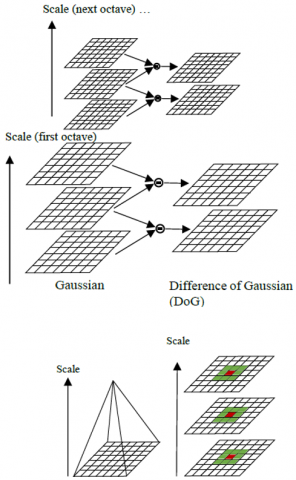Figure 1. A DoG pyramid 

\begin{aligned} D(x, y, \sigma) &=(G(x, y, k \sigma)-G(x, y, \sigma)) \otimes I(x, y) \\ &=L(x, y, k \sigma)-L(x, y, \sigma) \end{aligned}    (2)

where, k is a scale coefficient of an adjacent scale-space factor, I(x,y) is an input image and $\otimes$ is the convolution operation in x and y. An improved Gauss-Laplace method, the poorly localized along edges are rejected. Finally, the interpolation is deployed to locate the keypoint accurately in scale and space.

2.1.3 Orientation assignment

The orientation assignment is the most advantage of SIFT’s histogram-based approach. Where a direction to each keypoint based on local image gradients. Consequently, the orientation histograms are created for peaks in the histogram. Finally, a keypoint is assigned as multiple orientations. The direction to each keypoint and orientation histogram in term of Laplace Transform can be written as:

$m(x, y)=\sqrt{(L(x+1, y)-\mathrm{L}(x-1, y))^{2}+(L(x, y+1)-\mathrm{L}(x, y-1))^{2}}$

$\theta(x, y)=\tan ^{-1}((L(x, y+1)-\mathrm{L}(x, y-1)) /(L(x+1, y)-\mathrm{L}(x-1, y)))$    (3)

where, m(x,y) is a direction to each keypoint based on local image gradients and θ(x,y) is a 36-bin orientation histogram.

2.1.4 Feature description and matching

The satisfy keypionts of orientation assignment are compared with each other keypoints under the various robustness properties. For example, the 16-separate- orientation histograms have a 4x4 neighborhood around each keypoint. The histograms are calculated with respect to the keypoint scale and orientation, which have been districted in previous steps. Therefore, each histogram has 8 orientation bins. Since, the contents of all histograms are connected to a 128-element (16x8) vector. Therefore, the feature vector is the keypoint descriptor which is shown in Figure 2.

## 2.png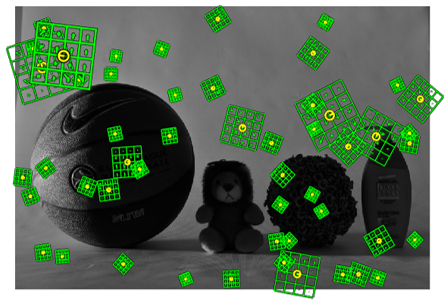Figure 2. Keypoint descriptors

In addition, the normalizing vector makes it more robust to changing illumination. Between the two feature vectors from mention image and unregistered one, Euclidean distance criterion is selected as the protocol to identify the matching field. This distance vectors can be written as:

Distance $(a, b)=\sqrt{\sum_{i=1}^{128}\left(a_{i}-b_{i}\right)^{2}}$    (4)

where, a and b (two-128 dimensional vectors) denote column vectors. In this criterion, if the distance value is lower than a certain ratio, it is corresponding point. This point regards to certain feature point as shown in Figure 3.

## 3.pngFigure 3. Feature matching 

The morphology approach for non-uniform illumination and other invariants consists of Top-Hat transformation and moment invariants.

2.2 Top-Hat transformation

Recently, Ye and Peng  proposed the main content of mathematical morphology so called Top-Hat transformation. This is the most desirable fundamental transformation which detects both of peak and valley on image gray values. The Top-Hat procedure consists of three operations, i.e., the determined of discrete functions, the utilization of morphological operations and the Top-Hat operation. The Top-Hat operator is separated into opening and closing Top-Hat operators. These operations can be written as:

OTHf,b(x) = (f - f ○ b)(x)    (5)

CTHf,b(x) = (f $\bullet$ f - f)(x)    (6)

Eqns. (5) and (6) are opening and closing Top-Hat operators, respectively. Where, f(x) and b(x) are discrete functions determined on discrete space F and B, respectively. Whilst, the opening () and closing ($\bullet$) morphological operations are utilized to f(x) and b(x), respectively.

2.3 Moment invariants

Especially, the invariant regards to scaling, translation and rotation. Hu moment invariants  have been extensively used to pattern recognition in many classification tasks. This moment invariant consists of slant and absolute orthogonal. The slant orthogonal is obtained from one slant orthogonal invariant based on algebraic invariants. Whilst, the absolute orthogonal is obtained from six absolute orthogonal invariant. These orthogonal invariants are not only independent of orientation, size and position but also maverick of parallel projection.

However, the pragmatic applications are noise-free and not continuous. Since images are quantized by finite-precision pixels in a discrete coordinate . For a two-dimensional image continuous function f(x,y) denotes piecewise-defined function, the order moment Mpq of order (p and q) is determined as:

$M_{p q}=\int_{-\alpha}^{\alpha} \int_{-\alpha}^{\alpha} x^{p} y^{q} f(x, y) d x d y$    (7)

where, p and q is 0,1,2,… accommodating this to scalar image. In term of pixel intensities I(x,y), the order moments Mij of pixel intensities are defined as:

$M_{i j}=\sum_{x} \sum_{y} x^{i} y^{j} I(x, y)$     (8)

As shown in Eq. (7) and (8), the moments may be not invariant when f(x,y) changes by scaling and rotating or translating. Therefore, the invariant features can be accomplished using central moments, which are determined as follows:

$\mu_{p q}=\int_{-\alpha}^{\alpha} \int_{-\alpha}^{\alpha}(x-\bar{x})^{p}(y-\bar{y})^{q} f(x, y) d x d y$     (9)

where, $\bar{x}$= M10/M00 and $\bar{y}$= M01/M00 are the centroid of the image f(x,y). And μpq is the centroid moments which is constructed using the centroid of the image f(x,y). Typically, this centroid moment is equivalent to the order moment. The center moment has been displaced to centroid of the image. Hence, the central moments are being unchanged under image translations. Huang and Leng  presented seven moment invariants where the scale invariance can be received by normalization. The normalize centroid moment can written as:

$\eta_{p q}=\frac{\mu_{p q}}{\mu_{o o} \times[1+(p+q) / 2]}$    (10)

where, p+q≥2 is translational invariance directly. Therefore, the normalized central moments are calculated as follows:

φ12002

φ2=(η2002)2 +4η112

φ3=(η30-3η12)2 +(3η2103)2

φ4=(η3012)2+(η2103)2

φ5=(η30+3η12)(η3012)[(η3012)2 -3(η2103)2] +(3η2103)(η2103)[3(η3012)2 - (η2103)2]

φ6=(η2002)[(η30+ η12)2 -(η21+ η03)2] +4η1130 + η12)(η21+ η03)

φ7=(3η21– η03)(η30 + η12)[(η30+ η12)2-3(η21+ η03)2]-(η30–3η12)(η21 + η03)[3(η30 + η12)2 -(η2103)2]    (11)

Eq. (11) shows the seven moment invariants which are not invariant under image scaling, rotation and translation.

3. The Proposed Method

As mention earlier, the keypoints were constructed by the moment invariants. This keypoint detection procedure can segment the targets from uneven illumination particle images. While the description was SIFT approach of two-dimensional image-based recognition for other invariants including rotation, viewpoint and illumination conditions. The central moments are being unchanged under image translations. In this section the proposed algorithm and experimental setup are presented.

3.1 The proposed algorithm

Figure 4 shows the proposed algorithm of a SIFT description approach for non-uniform illumination and other invariants, where the algorithm is implemented using the SIFT approach of keypoint detection and description steps.

As shown in Figure 4, the proposed algorithm using the SIFT approach based on three procedures, i.e., pre-processing procedures, keypoints detection procedure and keypoints description procedure. Firstly, the pre-processing procedure is pre-image processing and feature extraction. This procedure uses morphological operations , Top-Hat filtering and average filtering technique. These pre-processing is organized as follows:

 Step 1 Gray image: the set of images are acquired from camera. These images are converted to grayscale. Step 2 Reverse: the backgrounds are estimated by the morphological opening operations and Top-Hat filtering. The background is brighter in the center of the image. Step 3 Subtract background: background image is subtracted from original image. Step 4 Top-Hat filtering: average filtering technique is applied to remove the poor candidate keypoints background image. As mentioned earlier, an existing improved keypoint detection (IKD) algorithm for non-uniform illumination is IKDSIFT . Figure 5 particularly compares various results of the proposed algorithm to IKDSIFT approach. In an attempt to enable fair comparisons, all images are the same background. For purposes of information, irrelevant results of proposed algorithm as well as relevant results of removable the poor candidate keypoints. Step 5 Thresholding: the image creates a new binary image. Step 6 Color map matrix: by identifying objects in the image, a label matrix is created as a color map matrix.

## 4.png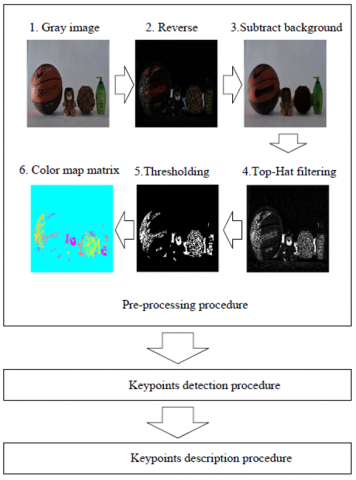Figure 4. Proposed algorithm

## 5.png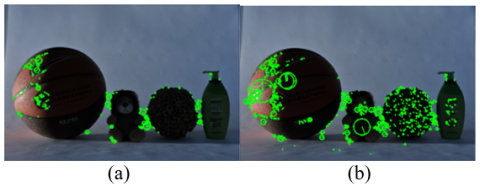Figure 5. Comparisons of (a) the proposed algorithm and (b) the existing IKDSIFT

3.2 Experimental setup

As a simple example, the data sets are provided by the Purdue RVL SPEC-DB and the PHOS. These data sets are available at https://engineering.purdue.edu/RVL/Database/specularity_database/. And the PHOS is available at http://utopia.duth.gr/~dchrisos/pubs/database2.html. Therefore, the color image database of data set is shown in Figure 6. This data set consists of three hundred images (150x150) and thirty images (851x566). These images are different illumination, viewpoint and rotation changes. On the other hand, the test and training set consist of one hundred and two hundred images, respectively. The confusion matrix is used to evaluate descriptors . This matrix based on the images including sensitivity rate, accuracy rate and precision rate of data sets. These rates can be written as:

$\%$ Sen $=\frac{T P}{T P+F N} \times 100$    (12)

$\% A c c=\frac{T P+T N}{T P+F N+T N+F P} \times 100$    (13)

$\%$ Prec $=\frac{T P}{T P+F P} \times 100$    (14)

where, %Sen%Acc and%Prec denote sensitivity rate or recall rate, accuracy rate and precision rate, respectively. It is possible for a keypoint to be true positive (TP) which is correctly identified. On the other hand, true negative (TN) which is correctly rejected. Consequently, FP and FN are incorrectly identified and incorrectly rejected, respectively.

All experiments were calculated on a 2.13GHz Intel(R) Core i5 CPU and 4GB RAM under Windows7. The proposed algorithm and its variants are implemented using C API that it was complied with VLFeat library .

## 6.png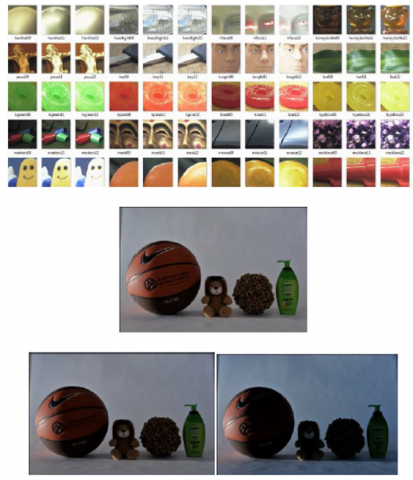Figure 6. An image set for investigating uniform and non-uniform illumination invariance

4. The Results and Discussion

The results and discussion of the proposed algorithm are presented in this section. This algorithm is constructed by using the SIFT method, Top-Hat filtering and morphological operations in pre-processing steps. Not only, differentiate between some of the particles and neighboring pixel, this technique can segment the targets from uneven illumination particle images.

4.1 The keypoint detection results

As mentioned earlier, the keypoint detections are typically based on the Scale Invariance Feature Transform (SIFT), an improved keypoint detection algorithm for non-uniform illumination (IKDSIFT), affine-SIFT (ASIFT) and proposed SIFT algorithm. Figure 7 particularly compares keypoints results of the proposed SIFT to those of existing SIFT approaches. In an attempt to enable fair comparisons, all images are the same conditions especially the non-uniform illumination situations.

## 7.pngFigure 7. The keypoint detection under non-uniform illumination. (a) the proposed (b) SIFT (c) ASIFT and (d) IKDSIFT

For purposes of information, irrelevant results of others SIFT techniques as well as relevant results of these techniques that are not fully homogenous are also included in Figure 8.

## 8.png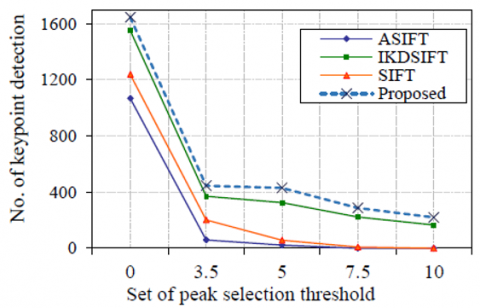Figure 8. The comparison of keypoint detection under non-uniform illumination

As shown in Figure 8, the maximum and minimum of keypoints detection are 1,645 and 220 keypoints, where are obtained by proposed algorithm. At the same peak selection threshold, the proposed SIFT highly detects the number of keypoints compared with the others conventional SIFT. Additionally, the proposed SIFT is significantly superior to the others conventional SIFTs.

4.2 The keypoint matching and description results

As mentioned earlier, keypoint matching and descriptions are typically based on SIFT, ASIFT or IKDSIFT. In an attempt to enable fair comparisons, all data set are fully homogenous. The data sets are provided by the Purdue RVL SPEC-DB and PHOS.

The keypoint matching and description results based on the images including sensitivity rate, accuracy rate and precision rate of data sets. The results show that the proposed can be matched as rotation, view point and illumination changes. The best result comparing to others under the invariants are obtained. These invariants are non-uniform versus non-uniform and non-uniform versus normal illumination. The evaluation of keypoints descriptors and matching under invariants are shown in Table 1. The high sensitivity, high accuracy and high precision rate of proposed are 100.00%, 83.33%, and 80.00%, respectively. Additionally, the proposed SIFT is highly desirable of sensitivity, accuracy and precision rate. Moreover, the proposed SIFT highly evaluate of keypoints descriptors and matching under invariants compared with the others conventional SIFT.

Table 1. To evaluate the keypoint descriptions and matching under invariants

 Evaluation Proposed SIFT ASIFT IKDSIFT %Sen 100.00% 75.00% 66.67% 100.00% %Acc 83.33% 66.67% 50.00% 83.33% %Pre 80.00% 75.00% 50.00% 75.00%

## 9.png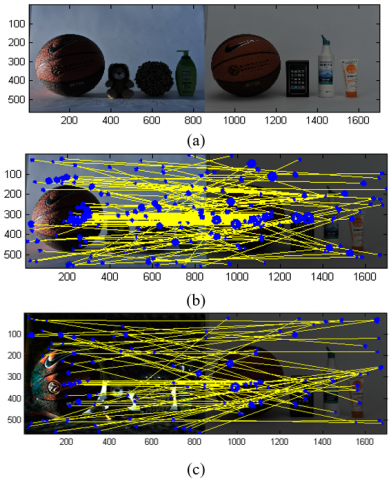Figure 9. The comparison of keypoint matching under viewpoint changes and non-uniform illumination. (a) Training and test dataset, (b) Our proposed and (c) IKDSIFT approach

In addition, as a simple example, the training and test dataset under the invariants such as non-uniform versus non-uniform and non-uniform versus normal illumination are displayed in Figure 9a. For purposes of information, irrelevant results of IKDSIFT techniques are also included in Figure 9b and 9c.

The number of keypoint matching which is correctly identified (TP) of 522 keypoints and incorrectly identified (FP) of 9 keypoints obtained by the proposed. Moreover, the proposed SIFT highly detects the number of keypoints compared with the IKDSIFT. Additionally, the proposed SIFT is significantly superior to IKDSIFT under the invariants.

5. Conclusion

In this article, a new improvement keypoint description algorithm of image-based recognition for other invariants is proposed. This proposed algorithm using the SIFT approach based on three procedures, i.e., pre-processing procedures, keypoints detection procedure and keypoints description procedure. The high sensitivity, high accuracy and high precision rate of proposed are 100.00%, 83.33%, and 80.00%, respectively. The proposed SIFT is highly desirable of sensitivity, accuracy and precision rate. Moreover, the proposed SIFT highly evaluate of keypoints descriptors and matching under invariants compared with the others conventional SIFT. Finally, the results show that the performance of the proposed is superior to the other SIFT in terms of non-uniform versus non-uniform and non-uniform versus normal illumination. For the future work, a new improvement keypoint description algorithm should be compared with the existing SIFT based on scale-free feature transformation.

Acknowledgment

The authors are grateful to Assoc.Prof. Dr. Anupap Meesomboon, Department of Electrical Engineering, Faculty of Engineering, Khon Kaen University, Khon Kaen, Thailand, for his useful suggestions.

References

 Kim, D., Rho, S., Hwang, E. (2012). Local feature-based multi-object recognition scheme for surveillance. Engineering Applications of Artificial Intelligence, 25(7): 1373-1380. https://doi.org/10.1016/j.engappai.2012.03.005

 Yong, K.P., Yi, L.Z., Xi, E.C., Yi, C.L., Shi, D.Z. (2018). An object detection method based on the joint feature of the H-S color descriptor and the SIFT feature. International Conference on Audio, Language and Image Processing (ICALIP), 14(8): 1-20. https://doi.org/10.1109/ICALIP.2018.8455641

 Kim, B., Yoo, H., Sohn, K. (2013). Exact order based feature descriptor for illumination robust image matching. Pattern Recognition, 46: 3268-3278. https://doi.org/10.1016/j.patcog.2013.04.015

 Li, D. (2010). Analysis of moment invariants on image scaling and rotation. In: Sobh T., Elleithy K. (eds) Innovations in Computing Sciences and Software Engineering, Springer, Dordrecht, 415-419. https://doi.org/10.1007/978-90-481-9112-3_70

 Wu, J., Cui, Z., Sheng, V.S., Zhao, P., Su, D., Gong, S. (2013). A comparative study of SIFT and its variants. Measurement Science Review, 13(3): 122-131. https://doi.org/10.2478/msr-2013-0021

 Hamdini, R., Diffellah, N., Namane, A. (2019). Robust local descriptor for color object recognition. Traitement du Signal, 36(6): 471-482. https://doi.org/10.18280/ts.360601

 Lowe, D.G. (2004). Distinctive image feature from scale-invariant keypoints. International Journal of Computer Vision, 60(2): 91-110. https://doi.org/10.1023/B:VISI.0000029664.99615.94

 Morel, J.M., Yu, G. (2013). ASIFT: A new framework for fully affine invariant image comparison. Imaging Sciences, 2(2): 438-469. https://doi.org/10.1137/080732730

 Wang, W.C., Cui, X.J. (2013). A segmentation method for uneven illumination particle images. Applied Sciences, Engineering and Technology, 5(4): 1284-1289. https://doi.org/10.19026/rjaset.5.4863

 Boonsivanon, K., Meesomboon, A. (2016). IKDSIFT: An improved keypoint detection algorithm based-on SIFT approach for non-uniform illumination. Procedia Computer Science, 86: 269-272. https://doi.org/10.1016/j.procs.2016.05.055

 Liao, K., Liu, G., Hui, Y. (2013). An improvement to the SIFT descriptor for image representation and matching. Pattern Recognition Letter, 34(11): 1211-1220. https://doi.org/10.1016/j.patrec.2013.03.021

 Kim, B., Yoo, H., Sohn, K. (2013). Exact order based feature descriptor for illumination robust image matching. Pattern Recognition, 46(12): 3268-3278, https://doi.org/10.1016/j.patcog.2013.04.015

 Chen, Y., Shang, L. (2016). Improved SIFT image registration algorithm on characteristic statistical distributions and consistency constraint. Optik, 127(2): 900-911. https://doi.org/10.1016/j.ijleo.2015.10.145

 Mao, W., Peng, X.W. (2019). WLIB-SIFT: A distinctive local image feature descriptor. IEEE 2nd International Conference on Information Communication and Signal Processing (ICICSP), pp. 379-383. https://doi.org/10.1109/ICICSP48821.2019.8958587

 Ye, B., Peng, J. (2002). Small target detection method based on morphology top-hat operator. Image. Gr., 5(5): 638-642.

 Huang, Z., Leng, J. (2010). Analysis of Hu's moment invariants on image scaling and rotation. Proceedings of 2010 2nd International Conference on Computer Engineering and Technology (ICCET), pp. 476-480. https://doi.org/10.1109/ICCET.2010.5485542

 Vedaldi, A., Fulkerson, B. (2010). VLFeat-An open and portable library of computer vision algorithms. Proceedings of the 18th International Conference on Multimedia (MM’10), Firenze, Italy. https://doi:10.1016/j.patrec.2013.03.021